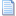1. ##2+2=?

If 2+2=fish what does 4x4=?

that is the first post (mine)
the next person to post answers the question and asks a random question of their own.Reply With Quote

2. ##Since n/n=1 and n/0=Infinity and 1=Infinity x 0, therefore n/n=Infinity x 0, n=nInfinityx0=0 therefore 2=0 and 4=0 therefore 4x4=0

Is the following statement true: This statement is false. ?Reply With Quote

3. ##Yes, because it is a delusional statement that completely believes that it is false, so it is being entirely truthful when it says that it is false.

Why?Reply With Quote

4. ##Reply With Quote

5. ##My spanish teacher showed ud that once! /off-topicReply With Quote

6. ##If 2+2= Fish, 4x4= Chips

If 2+2=Crap, 4x4=?Reply With Quote